# MultiFIT: Multivariate Multiscale Framework for Independence Tests

The MultiFit pcakage includes several functions, of which the most important are:
1. MultiFit: the function that runs the test of independence of two random vectors, the algorithm comprising of multiscale $$2\times2$$ univariate tests of discretized margins and multiple testing adjustments. At each resolution, recursively, the most significant tests are chosen and smaller portions of the sample space that correspond to those are explored in higher resolutions. The function returns a list object that contains details of the performed tests, adjusted p-values for all tests, and global p-values for the null hypothesis that the two random vectors are independent.
2. permNullTest: a function that, for a given data set and output of the MultiFit function, computes a permutation null distribution for test statistics for the global null hypothesis that are provided by MultiFit1.
3. multiSummary: a function that returns and plots the most significant $$2\times2$$ univariate tests of discretized margins.
4. multiTree: a function that generates a directed acyclic graph where nodes are $$2\times2$$ univariate tests of discretized margins. An edge from one test to another indicates the the latter test is performed on half the portion of the sample space on which the former was perofrmed.

The following demonstrates the usage of the above functions. For a full discussion of the framework see Gorsky and Ma (2018).

## Examples

### First Example:

#### Generate Data:

set.seed(1)
# Generate data for two random vectors, each of dimension 2, 300 observations:
n=300
x = matrix(0, ncol=2, nrow=n)
y = matrix(0, ncol=2, nrow=n)

# x1 and y1 are i.i.d Normal(0,1):
x[,1]=rnorm(n)
y[,1]=rnorm(n)

# x2 is a Uniform(0,1):
x[,2]=runif(n)

# and y2 is depends on x2 as a noisy sine function:
y[,2]=sin(5*pi*x[,2]) + 0.6*rnorm(n)

plot(x[,1],y[,1], col="grey", pch="x", xlab="x1", ylab="y1")
plot(x[,1],y[,2], col="grey", pch="x", xlab="x1", ylab="y2")
plot(x[,2],y[,1], col="grey", pch="x", xlab="x2", ylab="y1")
plot(x[,2],y[,2], col="grey", pch="x", xlab="x2", ylab="y2")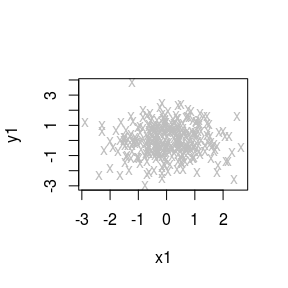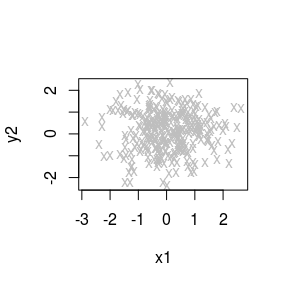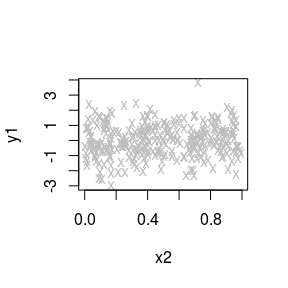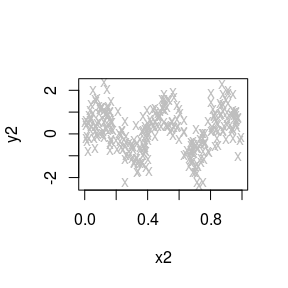#### Run the Test:

library(MultiFit)
fit = multiFit(x=x, y=y)
fit\$p.values
##            H   Hcorrected           MH
## 8.925850e-13 4.477071e-13 1.679095e-13

In order to get a better sense of the workings of the function, choose verbose=TRUE:

# Data may also be transferred to the function as a single list:
xy = list(x=x,y=y)
fit = multiFit(xy, verbose=TRUE)
## Applying rank transformation
## Testing and computing mid-p corrected p-values:
## Resolution 0/4: performing 4 tests
## Resolution 1/4: scanning 16 windows, performing 32 tests
## Resolution 2/4: scanning 40 windows, performing 120 tests
## Resolution 3/4: scanning 40 windows, performing 128 tests
## Resolution 4/4: scanning 40 windows, performing 144 tests
## Time difference of 0.03971839 secs
## Individual tests completed, post-processing...
## H: Computing all adjusted p-values...
## Hcorrected: Computing all adjusted p-values...
## MH: Computing CDF...
## MH: Computing all adjusted p-values...
## Time difference of 0.001939058 secs
##
## Fisher's Exact Tests:
## Mean of -log(p-values): 1.4406
## Mean of top 4 -log(p-values): 17.1992
## Mean of -log(p-values with mid-p correction): 1.64465
## Mean of top 4 -log(p-values with mid-p correction): 17.7277
##
## Global p-value, Holm on p-values: 8.92585e-13
## Global p-value, Holm on p-values with mid-p correction: 4.47707e-13
## Global p-value, Modified Holm step-down: 1.67909e-13
The output details the number of tests performed at each resolution. The default testing method for the marginal $$2\times2$$ contingency tables is Fisher’s exact test. Several global test statistics are reported:
• Mean of $$-log(\text{p-values})$$
• Mean of top 4 $$-log(\text{p-values})$$
• Mean of $$-log(\text{p-values with mid-p correction})$$
• Mean of top 4 $$-log(\text{p-values with mid-p correction})$$).

These are not associated with p-values until we genrate a permutation null distribution for them using permNullTest. The default multiple testing adjustments methods we use are Holm’s method on the original p-values (H), Holm’s method on the mid-p corrected p-values (Hcorrected) and a Modified Holm (MH)2. The p-value for the global null hypothesis that $$\mathbf{x}$$ is independent of $$\mathbf{y}$$ is reported for each adjustment method.

#### Summarize Results (1):

In order to get a sense of the specific marginal tests that are significant at the alpha=0.005 level, we may use the function multiSummary:

multiSummary(xy=xy, fit=fit, alpha=0.005)
##
## The following tests had a p-value of less than 0.005:
## Ranked #1, Test 152: x2 and y2 | 0.46<=x2<0.74 (p-value=1.679095e-13)
## Ranked #2, Test 32: x2 and y2 | 0<=x2<0.46 (p-value=3.112791e-05)
## Ranked #3, Test 168: x2 and y2 | -2.89<=x1<-0.04, 0<=x2<0.46, -3.01<=y1<-0.04 (p-value=0.003069073)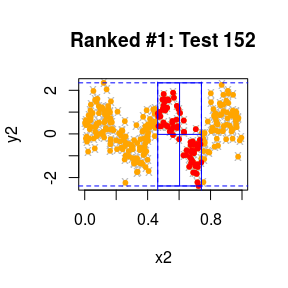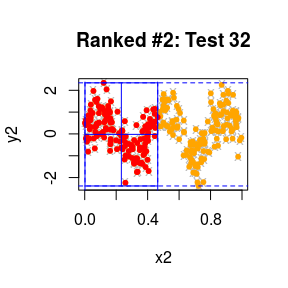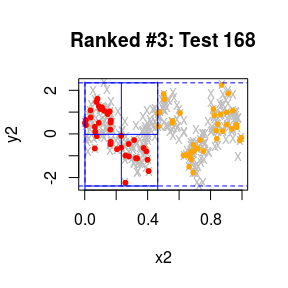In grey and orange are all data points outside the window we are testing. In orange are the points that were in the window if we were not to condition on the margins that are visible in the plot. In red are the points that are inside the window after we condition on all the margins, including those that are visible in a plot. The blue lines delineate the quadrants along which the discretization was performed: we count the number of red points in each quadrant, treat these four numbers as a $$2\times2$$ contingency table and peform a 1-degree of freedom test of independence on it (default test: FIsher’s exact test).

#### Summarize Results (2):

We may also draw a directed acyclic graph where nodes represent tests as demonstrated above in the multiSummary output. An edge from one test to another indicates that the latter test is performed on half the portion of the sample space on which the former was perofrmed. Larger nodes correspond to more exteme p-values for the test depicted in it (storing the output as a pdf file):

# And plot a DAG representation of the ranked windows:
library(png)
library(qgraph)
multiTree(xy=xy, fit=fit, filename="first_example")
## Output stored in /tmp/RtmpRDbEZw/Rbuild3de743b2798a/MultiFit/vignettes/first_example.pdf

We see that, in agreement with the output of the multiSummary function, nodes 152, 32 and 168 (which correspond to tests 152, 32 and 168) are the largest compared to the other nodes.

#### Global Test with a Permutation Null:

We may also generate a permutation null distribution for global the test statistics that are shown in the output of MultiFit and compute the respective p-values using permNullTest and specifying the size of the simulated null distribution with the parameter perm.null.sim.

perm.null.first_example = permNullTest(perm.null.sim=1000L, xy=xy, fit=fit,
verbose=TRUE)

#### Test More Windows:

In the defualt setting, M, the number of top ranking tests per resolution that will be further explored in higher resolutions, is set to 10. We may choose, e.g., M=100, which takes longer. In this case the MultiFit identifies more windows with adjusted p-values that are below alpha=0.005. However, the global adjusted p-values are less extreme than when performing the MultiFit with fewer tests:

fit100 = multiFit(xy, M=100, verbose=TRUE)
## Applying rank transformation
## Testing and computing mid-p corrected p-values:
## Resolution 0/4: performing 4 tests
## Resolution 1/4: scanning 16 windows, performing 32 tests
## Resolution 2/4: scanning 128 windows, performing 160 tests
## Resolution 3/4: scanning 400 windows, performing 608 tests
## Resolution 4/4: scanning 400 windows, performing 1108 tests
## Time difference of 0.07389736 secs
## Individual tests completed, post-processing...
## H: Computing all adjusted p-values...
## Hcorrected: Computing all adjusted p-values...
## MH: Computing CDF...
## MH: Computing all adjusted p-values...
## Time difference of 0.004466772 secs
##
## Fisher's Exact Tests:
## Mean of -log(p-values): 1.17249
## Mean of top 4 -log(p-values): 22.0729
## Mean of -log(p-values with mid-p correction): 1.39328
## Mean of top 4 -log(p-values with mid-p correction): 22.6908
##
## Global p-value, Holm on p-values: 2.7563e-12
## Global p-value, Holm on p-values with mid-p correction: 1.38252e-12
## Global p-value, Modified Holm step-down: 2.04683e-13
multiSummary(xy=xy, fit=fit100, alpha=0.005, plot.tests=FALSE)
##
## The following tests had a p-value of less than 0.005:
## Ranked #1, Test 192: x2 and y2 | 0.46<=x2<0.74 (p-value=2.046834e-13)
## Ranked #2, Test 584: x2 and y2 | 0.46<=x2<0.74, -3.01<=y1<-0.04 (p-value=5.611506e-08)
## Ranked #3, Test 344: x2 and y2 | -2.89<=x1<-0.04, 0.46<=x2<0.74 (p-value=3.471186e-06)
## Ranked #4, Test 480: x2 and y2 | -0.04<=x1<2.65, 0.46<=x2<0.74 (p-value=4.289188e-05)
## Ranked #5, Test 32: x2 and y2 | 0<=x2<0.46 (p-value=7.752383e-05)
## Ranked #6, Test 120: x2 and y2 | 0<=x2<0.46, -3.01<=y1<-0.04 (p-value=0.0001482809)
## Ranked #7, Test 680: x2 and y2 | 0.46<=x2<0.74, -0.04<=y1<3.81 (p-value=0.0006539169)

In order to perform the test even more exhaustively, one may:

# 1. set M=Inf, running through all windows up to the maximal resolution
# which by default is set to log2(n/100):
ex1 = multiFit(xy, M=Inf)

# 2. set both M=Inf and the maximal resolution max.res=Inf.
# In this case, the algorithm will scan through higher and higher resolutions,
# until there are no more windows that satisfy the minimum requirements for
# marginal totals: min.win.tot, min.row.tot and min.col.tot (whose default values
# are presented below):
ex2 = multiFit(xy, M=Inf, max.res=Inf,
min.win.tot = 25L, min.row.tot = 10L, min.col.tot = 10L)

# 3. set smaller minimal marginal totals, that will result in testing
# even more windows in finer resolutions:
ex3 = multiFit(xy, M=Inf, max.res=Inf,
min.win.tot = 10L, min.row.tot = 4L, min.col.tot = 4L)

### A Local Signal:

MultiFit excels in locating very localized signals.

#### Generate a Local Signal:

# Generate data for two random vectors, each of dimension 2, 800 observations:
n=800
x = matrix(0, ncol=2, nrow=n)
y = matrix(0, ncol=2, nrow=n)

# x1, x2 and y1 are i.i.d Normal(0,1):
x[,1]=rnorm(n)
x[,2]=rnorm(n)
y[,1]=rnorm(n)

# y2 is i.i.d Normal(0,1) on most of the space:
y[,2]=rnorm(n)
# But is linearly dependent on x2 in a small portion of the space:
w=rnorm(n)
portion.of.space = x[,2]>0 & x[,2]<0.7 & y[,2]>0 & y[,2]<0.7
y[portion.of.space,2] = x[portion.of.space,2]+(1/12)*w[portion.of.space]
xy.local = list(x=x, y=y)

#### Search for It and Summarize the Results:

Truly local signals may not be visible to our algorithm in resolutions that are lower than the one that the signal is embedded in. In order to cover all possible tests up to a given resolution (here: resolution 4), we use the parameter full.coverage.res=4 (from resolution 5 onwards, only the top M windows will be further tested):

fit.local = multiFit(xy=xy.local, full.coverage.res=4, verbose=TRUE)
## Applying rank transformation
## Testing and computing mid-p corrected p-values:
## Resolution 0/6: performing 4 tests
## Resolution 1/6: scanning 16 windows, performing 32 tests
## Resolution 2/6: scanning 128 windows, performing 160 tests
## Resolution 3/6: scanning 640 windows, performing 640 tests
## Resolution 4/6: scanning 2560 windows, performing 2240 tests
## Resolution 5/6: scanning 40 windows, performing 156 tests
## Resolution 6/6: scanning 40 windows, performing 108 tests
## No pairs of margins in resolution 6 had enough observations to be tested.
## Time difference of 0.2017508 secs
## Individual tests completed, post-processing...
## H: Computing all adjusted p-values...
## Hcorrected: Computing all adjusted p-values...
## MH: Computing CDF...
## MH: Computing all adjusted p-values...
## Time difference of 0.02344441 secs
##
## Fisher's Exact Tests:
## Mean of -log(p-values): 0.816647
## Mean of top 4 -log(p-values): 9.83624
## Mean of -log(p-values with mid-p correction): 0.998856
## Mean of top 4 -log(p-values with mid-p correction): 10.2239
##
## Global p-value, Holm on p-values: 1.56826e-05
## Global p-value, Holm on p-values with mid-p correction: 7.87512e-06
## Global p-value, Modified Holm step-down: 5.58324e-06
multiSummary(xy=xy.local, fit=fit.local, plot.margin=TRUE, pch="")
##
## The following tests had a p-value of less than 0.05:
## Ranked #1, Test 2928: x2 and y2 | -0.06<=x2<0.7, -0.06<=y2<0.62 (p-value=5.583243e-06)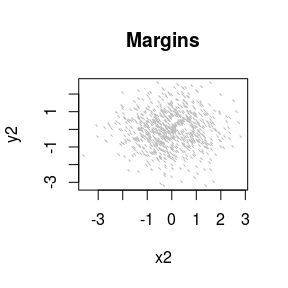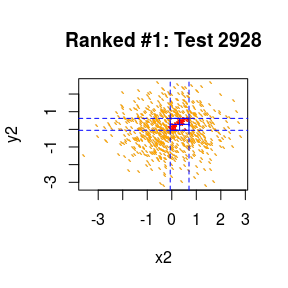### A Signal that is Spread Between More than 2 Margins:

MultiFit also has the potential to identify complex conditional dependencies in multivariate signals.

#### Generate Data and Examine Margins:

Take $$\mathbf{x}$$ and $$\mathbf{y}$$ to be each of three dimensions, with 700 data points. We first generate a marginal circle dependency: $$x_1$$, $$y_1$$, $$x_2$$, and $$y_2$$ are all i.i.d standard normals. Take $$x_3=\cos(\theta)+\epsilon$$, $$y_3=\sin(\theta)+\epsilon'$$ where $$\epsilon$$ and $$\epsilon'$$ are i.i.d $$\mathrm{N}(0,(1/10)^2)$$ and $$\theta\sim \mathrm{Uniform}(-\pi,\pi)$$. I.e., the original dependency is between $$x_3$$ and $$y_3$$.

# Marginal signal:

# Generate data for two random vectors, each of dimension 3, 800 observations:
n=800
x = matrix(0, ncol=3, nrow=n)
y = matrix(0, ncol=3, nrow=n)

# x1, x2, y1 and y2 are all i.i.d Normal(0,1)
x[,1]=rnorm(n)
x[,2]=rnorm(n)
y[,1]=rnorm(n)
y[,2]=rnorm(n)

# x3 and y3 form a noisy circle:
theta = runif(n,-pi,pi)
x[,3] = cos(theta) + 0.1*rnorm(n)
y[,3] = sin(theta) + 0.1*rnorm(n)

plot(x[,2],y[,2], col="grey", pch="x", xlab="x2", ylab="y2")
plot(x[,2],y[,3], col="grey", pch="x", xlab="x2", ylab="y3")
plot(x[,3],y[,2], col="grey", pch="x", xlab="x3", ylab="y2")
plot(x[,3],y[,3], col="grey", pch="x", xlab="x3", ylab="y3")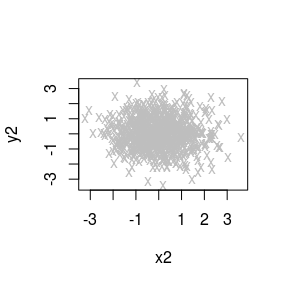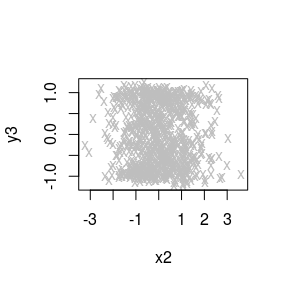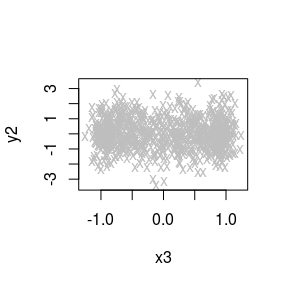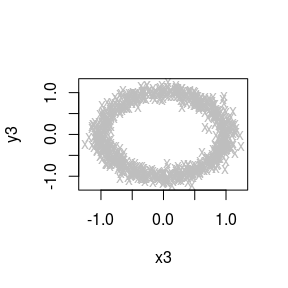Next, rotate the circle in $$\pi/4$$ degrees in the $$x_2$$-$$x_3$$-$$y_3$$ space by applying:

$$\left[\begin{matrix}\cos(\pi/4) & -sin(\pi/4) & 0\\\sin(\pi/4) & cos(\pi/4) & 0\\ 0 & 0 & 1\end{matrix}\right]\left[\begin{matrix}| & | & |\\X_2 & X_3 & Y_3\\| & | & |\end{matrix}\right]$$

I.e., once rotated the signal is ‘spread’ between $$x_2$$, $$x_3$$ and $$y_3$$, and harder to see through the marginal plots.

# And now rotate the circle:
phi = pi/4
rot.mat = matrix(c(cos(phi), -sin(phi),  0,
sin(phi),  cos(phi),  0,
0,         0,         1), nrow=3, ncol=3)
xxy = t(rot.mat%*%t(cbind(x[,2],x[,3],y[,3])))

x.rtt = matrix(0, ncol=3, nrow=n)
y.rtt = matrix(0, ncol=3, nrow=n)

x.rtt[,1]=x[,1]
x.rtt[,2]=xxy[,1]
x.rtt[,3]=xxy[,2]
y.rtt[,1]=y[,1]
y.rtt[,2]=y[,2]
y.rtt[,3]=xxy[,3]

plot(x.rtt[,2],y.rtt[,2], col="grey", pch="x", xlab="x2", ylab="y2")
plot(x.rtt[,2],y.rtt[,3], col="grey", pch="x", xlab="x2", ylab="y3")
plot(x.rtt[,3],y.rtt[,2], col="grey", pch="x", xlab="x3", ylab="y2")
plot(x.rtt[,3],y.rtt[,3], col="grey", pch="x", xlab="x3", ylab="y3")

xy.rtt.circ = list(x=x.rtt, y=y.rtt)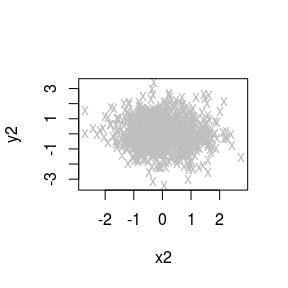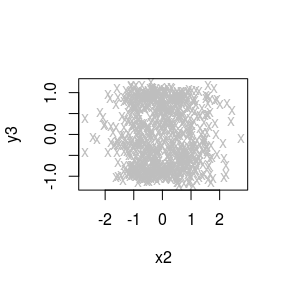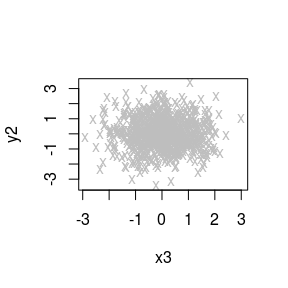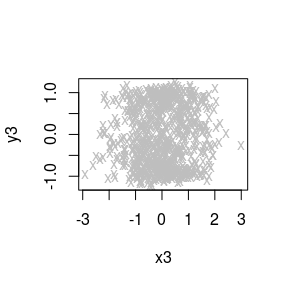#### Run the Test and Summarize the Data:

# This time, try another testing method, chi^2 (faster than the default Fisher's exact test, slightly less powerful):
fit.rtt.circ = multiFit(xy=xy.rtt.circ, test.method="chi.sq", verbose=TRUE)
## Applying rank transformation
## Testing and computing Yates corrected p-values:
## Resolution 0/6: performing 9 tests
## Resolution 1/6: scanning 36 windows, performing 108 tests
## Resolution 2/6: scanning 40 windows, performing 315 tests
## Resolution 3/6: scanning 40 windows, performing 252 tests
## Resolution 4/6: scanning 40 windows, performing 297 tests
## Resolution 5/6: scanning 40 windows, performing 315 tests
## Resolution 6/6: scanning 40 windows, performing 324 tests
## Time difference of 0.0567975 secs
## Individual tests completed, post-processing...
## H: Computing all adjusted p-values...
## Hcorrected: Computing all adjusted p-values...
## Time difference of 0.0003399849 secs
##
## Chi Squared Tests:
## Mean of -log(p-values): 1.26815
## Mean of top 4 -log(p-values): 18.9704
## Mean of -log(p-values with Yates correction): 0.997961
## Mean of top 4 -log(p-values with Yates correction): 17.8953
##
## Global p-value, Holm on p-values: 1.5366e-07
## Global p-value, Holm on p-values with Yates correction: 3.81007e-07
multiSummary(xy=xy.rtt.circ, fit=fit.rtt.circ, only.rk=1:4)
##
## The following tests had a p-value of less than 0.05:
## Ranked #1, Test 333: x3 and y3 | -2.7<=x2<-0.07, -1.23<=y3<-0.11 (p-value=3.810075e-07)
## Ranked #2, Test 837: x3 and y3 | -0.65<=x2<-0.07, -2.91<=x3<0.01, -0.11<=y3<1.23 (p-value=2.216985e-05)
## Ranked #3, Test 267: x2 and y3 | 0.01<=x3<3.01, -1.23<=y3<-0.11 (p-value=9.197692e-05)
## Ranked #4, Test 342: x3 and y3 | -2.7<=x2<-0.07, -0.11<=y3<1.23 (p-value=0.0001063328)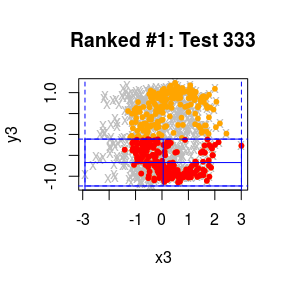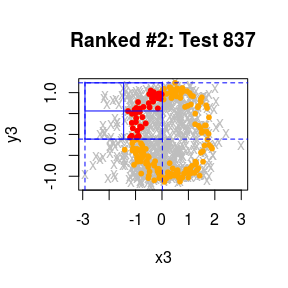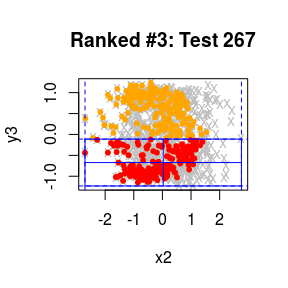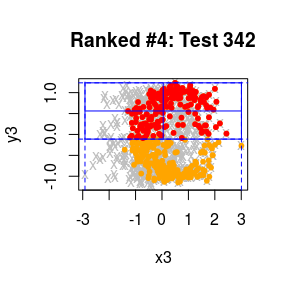Notice how the signal is detected both in the $$x_3$$-$$y_3$$ plane and the $$x_2$$-$$y_3$$ plane.

### A Superimposed Signal:

Here we examine MultiFit’s ability to
1. detect a signal that is comprised of two sine waves in different frequencies
2. given a third dimension that determines which data points belong to which wave, to see if our algorithm successfully identifies this relation.

We take $$\mathbf{x}$$ to be a two dimensional random variable and $$\mathbf{y}$$ to be one dimensional, all with 550 data points. Define $$x_1\sim U(0,1)$$, $$x_2\sim Beta(0.3,0.3)$$ independent of $$x_1$$, and define:

$$Y = \begin{cases} \sin(10\cdot x_1) + \epsilon, & \text{if }x_2 > 0.75\\ \sin(40\cdot x_1) + \epsilon, & \text{if }x_2\leq0.75 \end{cases}$$

#### Generate the Signal:

n=550
x=matrix(0,nrow=n,ncol=2)
x[,1]=runif(n)
x[,2]=rbeta(n,.3,.3)

epsilon=rnorm(n,0,0.3)

y=matrix(0,nrow=n,ncol=1)
y[,1]=sin(10*x[,1])*(x[,2]>0.75)+sin(40*x[,1])*(x[,2]<=0.75)+epsilon

plot(x[,1],y[,1], col="grey", pch="x", xlab="x2", ylab="y2")
plot(x[,2],y[,1], col="grey", pch="x", xlab="x2", ylab="y2")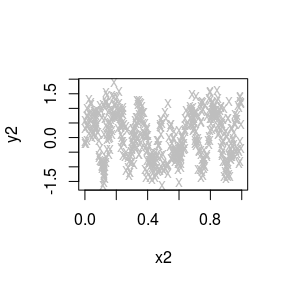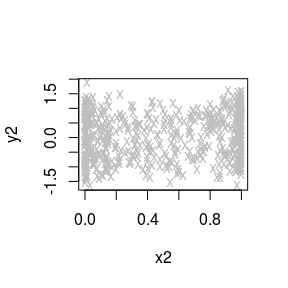#### Test and Summarize:

fit.superimpose=multiFit(x=x, y=y, M=100)
multiSummary(x=x, y=y, fit=fit.superimpose, alpha=0.0001)
##
## The following tests had a p-value of less than 1e-04:
## Ranked #1, Test 109: x1 and y1 | 0<=x1<0.48, 0.94<=x2<1 (p-value=1.330405e-06)
## Ranked #2, Test 3: x1 and y1 | 0<=x1<0.48 (p-value=1.336168e-06)
## Ranked #3, Test 25: x1 and y1 | 0<=x1<0.48, 0.48<=x2<1 (p-value=1.439459e-06)
## Ranked #4, Test 121: x1 and y1 | 0.48<=x1<0.76, 0.48<=x2<1 (p-value=5.577122e-06)
## Ranked #5, Test 387: x1 and y1 | 0.62<=x1<0.76, 0<=x2<0.48 (p-value=1.533995e-05)
## Ranked #6, Test 133: x1 and y1 | 0.76<=x1<1, 0.48<=x2<1 (p-value=6.686604e-05)
## Ranked #7, Test 427: x1 and y1 | 0.88<=x1<1, 0<=x2<0.48 (p-value=8.800438e-05)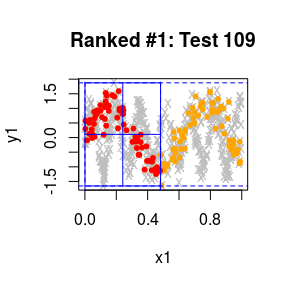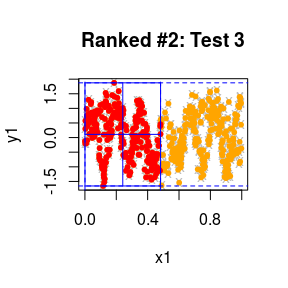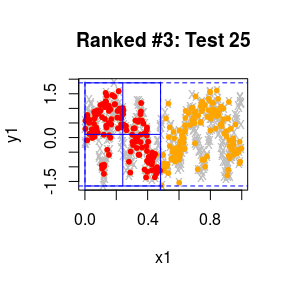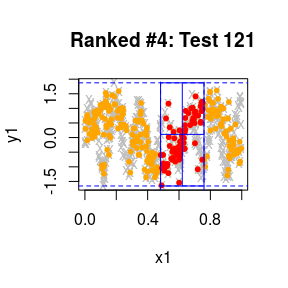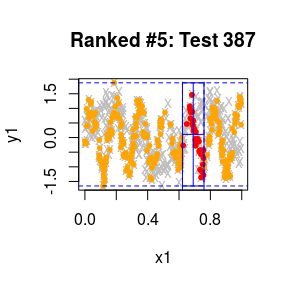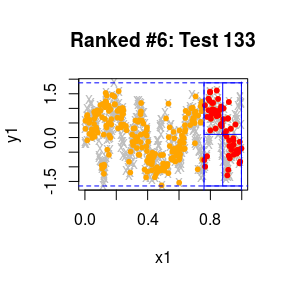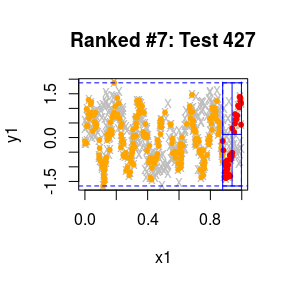Notice how the seperate signals are identified in the 1$$^{st}$$ and 5$$^{th}$$ ranking tests.

### A Univariate Example:

In the univariate case, Ma and Mao (2017) show that the p-values for Fisher’s exact test are mutually independent under the null hypothesis of independence. Thus, we may also generate approximate and theoretical null distributions for the global test statistics that are much faster to compute, compared to the permutation null distribution.

#### Generate Data:

n=300
# y is a noisy quadratic function of x:
x.uv = runif(n)
y.uv = (x.uv-0.5)^2 + 0.2*rnorm(n)

plot(x.uv,y.uv, col="grey", pch="x", xlab="x", ylab="y")

xy.uv = list(x=x.uv, y=y.uv)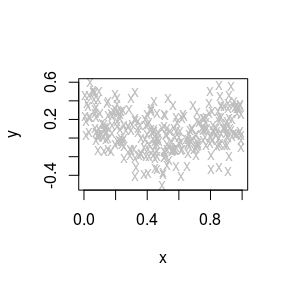#### Test:

# Apply the test and in addition compute approximate and theoretical null distributions for the global test statistics:
fit.uv = multiFit(xy=xy.uv, uv.approx.null = TRUE, uv.exact.null = TRUE,
uv.null.sim = 10000L, verbose=TRUE)
## Applying rank transformation
## Testing and computing mid-p corrected p-values:
## Resolution 0/4: performing 1 tests
## Resolution 1/4: scanning 4 windows, performing 4 tests
## Resolution 2/4: scanning 16 windows, performing 12 tests
## Resolution 3/4: scanning 40 windows, performing 28 tests
## Resolution 4/4: scanning 40 windows, performing 36 tests
## Time difference of 0.02278566 secs
## Individual tests completed, post-processing...
## H: Computing all adjusted p-values...
## Hcorrected: Computing all adjusted p-values...
## MH: Computing CDF...
## MH: Computing all adjusted p-values...
## Time difference of 0.000638485 secs
## Simulating an approximate null distribution...
## Time difference of 0.3708971 secs
## Simulating from the theoretical null distribution...
## Time difference of 2.157449 secs
##
## Fisher's Exact Tests:
## Mean of -log(p-values): 1.82851
## Mean of top 4 -log(p-values): 6.23007
## Mean of -log(p-values with mid-p correction): 2.10252
## Mean of top 4 -log(p-values with mid-p correction): 6.59663
##
## Global p-value, theoretical null, mean -log(p-values with mid-p correction): 0
## Global p-value, theoretical null, mean top 4 -log(p-values with mid-p correction): 0
##
## Global p-value, approximate null, mean -log(p-values with mid-p correction): 0
## Global p-value, approximate null, mean top 4 -log(p-values with mid-p correction): 0
##
## Global p-value, Holm on p-values: 0.00433626
## Global p-value, Holm on p-values with mid-p correction: 0.0026193
## Global p-value, Modified Holm step-down: 0.00205754

## Important Parameters for MultiFit:

• M: A positive integer (or Inf), the number of top ranking windows to continue to test at each resolution.
• max.res: A positive integer (or Inf), the maximal number of resolutions to scan (algorithm will stop at a lower resolution if all windows in it do not meet the criteria specified at min.win.tot, min.row.tot and min.col.tot. The default value for the max.res is $$\lfloor\log_2(n/10)\rfloor$$ where $$n$$ is the number of observations in the vectors.
• full.coverage.res: A positive integer, if set to an integer between 0 and max.res, all tests up to and including resolution full.coverage.res will be performed (algorithm will stop at a lower resolution than requested if all windows in it do not meet the criteria specified at min.win.tot, min.row.tot and min.col.tot). For higher resolutions the children of the top M ranking windows will be selected.
• rank.transform: Logical, if TRUE, marginal rank transform is performed on all margins of $$\mathbf{x}$$ and . If FALSE, all margins are scaled to 0-1 scale. When FALSE, the average and top statistics of the negative logarithm of the p-values are only computed for the univariate case.
• test.method: String, choose “Fisher” for Fisher’s exact test (slowest), “chi.sq” for $$\chi^2$$ test, “LR” for likelihood-ratio test and “norm.approx” for approximating the hypergeometric distribution with a normal distribution (fastest).
• correct: Logical, if TRUE compute mid-p corrected p-values for Fisher’s exact test, or Yates corrected p-values for the $$\chi^2$$ test, or Williams corrected p-values for the likelihood-ratio test.
• min.win.tot: Non-negative integer, the minimal number of observations per window below which a p-value for a given window will not be computed.
• min.row.tot: Non-negative integer, the minimal number of observations for row totals in the $$2\times2$$ contingency tables below which a contingency table will not be tested.
• min.col.tot: Non-negative integer, the minimal number of observations for column totals in the $$2\times2$$ contingency tables below which a contingency table will not be tested.
• p.adjust.methods: String, choose between "H" for Holm, "Hcorrected" for Holm with the correction as specified in correct, or "MH" for Modified-Holm (for Fisher’s exact test only).
• compute.all.holm: Logical, if FALSE, only global p-value is computed (may be faster, especially when Modified-Holm correction is used). If TRUE adjusted p-values are computed for all tests.
• cutoff: Numerical between 0 and 1, an upper limit for the p-values that are to be adjusted (the lower the cutoff - the fewer computations are required for the Modified Holm method).
• top.max.ps: Positive integer, report the mean of the top top.max.ps order statistics of the negative logarithm of all p-values.
• uv.approx.null: Logical, in a univariate case, if TRUE and the testing method is either Fisher’s exact test or the normal approximation of the hypergeometric distribution, an approximate null distribution for the global test statistics is simulated.
• uv.exact.null: Logical, in a univariate case, if TRUE and the testing method is either Fisher’s exact test or the normal approximation of the hypergeometric distribution, a theoretical null distribution for the global test statistics is simulated.
• uv.null.sim`: Positive integer, the number of simulated values to be computed in a univariate case when a theoretical or approximate null distribution is simulated.

1. The test based on the global test statistics may be more powerful for the global null hyppthesis, but does not provide adjusted p-values.

2. The latter is the most powerful and computationaly intense of the three